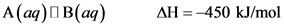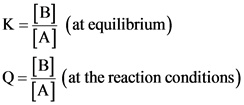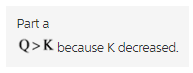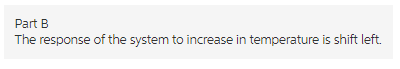# Consider this system at equilibrium.A (aq) <---> B (aq) deltaH - 450 kj/mol

Consider this system at equilibrium.
A (aq) <—> B (aq) deltaH - 450 kj/mol

What can be said about Q and K immediately after an increase in temperature?

Q > K because Q increased
Q > K because K decreased
Q< K bc Q decreased
Q< K bc K increased
Q= K bc neither changed

How will the system respond to a temperature increase?

shift left
shift right
no change

Concepts and reason
According to Le-Chatelier’s principle, any system at equilibrium is subjected to a change in volume, temperature, or pressure, the equilibrium shifts in a direction that minimizes the change and a new equilibrium is established.
For endothermic reactions, increase in temperature shifts the equilibrium to right side.
For exothermic reactions, increase in temperature shifts the equilibrium to left side.
At equilibrium, Q = K

Fundamentals
The reaction quotient, Q is the ratio of product of concentrations of products to the product of concentrations of reactants at the reaction conditions.
The equilibrium constant, K is the ratio of product of concentrations of products to the product of concentrations of reactants at equilibrium.
At equilibrium, Q = K

If Q < K, the reaction takes place in forward direction to reach the equilibrium.
If Q > K, the reaction takes place in backward direction to reach the equilibrium.

Part a
Consider the following reaction at equilibrium:Here, the change in enthalpy has negative sign. Hence, the reaction is exothermic.
The expressions for equilibrium constant, K and the reaction quotient, Q are as follows:If the temperature increases, the equilibrium shifts in a direction to minimize the change in temperature. The reaction absorbs the heat in back ward direction.

Therefore,Q > K because K decreased.Explanation:
As the temperature increases, the system should reach a new equilibrium in which the concentration of reactants increases. Hence, the K decreases.

Part b
The response of the system to increase in temperature is shift left.Explanation:
If the temperature increases, the equilibrium shifts in a direction to minimize the change in temperature. The reaction absorbs the heat in back ward direction.